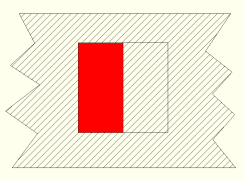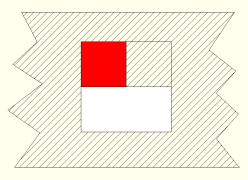# Alpha Premultiplication

February 15, 2003

When storing an RGBA image people often wonder whether to store the color components premultiplied by the alpha. For example, if you’ve got a pixel that’s full red (255,0,0) and has a 50% alpha (128), should the RGBA values store premultiplied colors (128,0,0,128) or non-premultiplied colors (255,0,0,128).

There are advantages to both schemes. For example, if you’re using non-premultiplied colors, then you can change the opacity of an image simply by tweaking its alpha channel. Or if you want to perform some color correction, say remap bright red to some other color, then you can do that without worrying that the alpha channel has made your bright red look like dark red simply because of transparency.

But if you’re using premultiplied colors, then the math of compositing images together is simpler and can avoid a division most of the time.

The key to understanding this is to give up the idea of alpha as transparent colored glass and instead see it as a screen mesh. So if your alpha is 50%, then imagine a pixel where half is covered by your pixel’s color and the other half is completely transparent. Specifically, imagine the left half covered and the right half transparent:Now the question is whether the RGB values should represent the color of the left half, or only the left half’s contribution to the whole pixel. The advantages of doing the former (non-premultiplied) were outlined above. To see that advantages of premultiplied colors, consider compositing another pixel on top of our half-red one. The new one is white (255,255,255) and 50% transparent. So since we’re using premultiplied colors, our RGBA is (128,128,128,128).

We’ve got this screendoor transparency model, but we don’t know where this half-pixel is compared to the half pixel we’re compositing over. If our new pixel is also on the left side, then we’ll clobber the underlying pixel altogether. If it’s on the right, we’ll patch the hole perfectly. Since we don’t know, we’ll go half-way and turn it 90 degrees, with the bottom half of the pixel opaque white and the top half transparent:So what do you see when you put the white pixel (foreground) on top of the red one (background)? The bottom half is white, the top-left quarter is red, and the top-right quarter is transparent:The math for that is easy when the numbers are premultiplied:

R' = RF + (1 - AF)×RB
G' = GF + (1 - AF)×GB
B' = BF + (1 - AF)×BB
A' = AF + (1 - AF)×AB

The result is (192,128,128,192), which makes sense if you think of each color’s contribution to the pixel times its area.

Now the math for non-premultiplied colors is hairier:

R' = (RF×AF + (1 - AF)×RB×AB) / A'
G' = (GF×AF + (1 - AF)×GB×AB) / A'
B' = (BF×AF + (1 - AF)×BB×AB) / A'
A' = AF + (1 - AF)×AB

You don’t need the divide if the foreground or the background is opaque, which is a common situation since people usually start with a fully-opaque image and add things to it.

If you’re using 8-bit colors, then premultiplying might make you lose a bunch of color precision that you’ll need later when you divide out the alpha to do some color correction. So I’d recommend not premultiplying colors if you care about accuracy and precision and have the time to waste during compositing. But premultiplying is better if you’ll be doing lots of compositing and you don’t need complete accuracy during color correction and blurs and such.

Either way, be aware of which scheme you’re using and think through each pixel operation so that the math makes sense given this screendoor transparency model.